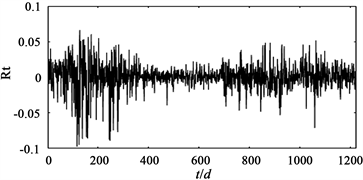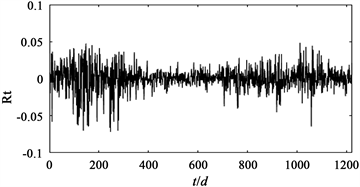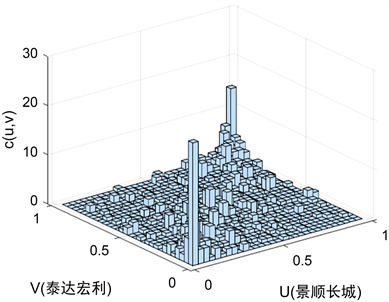﻿ 开放式基金投资组合风险度量——基于Copula-ARMA-GARCH模型

# 开放式基金投资组合风险度量——基于Copula-ARMA-GARCH模型The Risk Measurement on Portfolio of Open-End Fund—Based on Copula-ARMA-GARCH Model

Abstract: The Copula-ARMA-GARCH model is constructed to study the risk measurement on portfolio of open-end funds. The selected data are the daily unit net worth series of Invesco Great Wall and Teda Manulife. The ARMA-GARCH-t model is used to fit the edge distribution, and t-Copula function with good fitting effect is selected to describe the correlation structure between assets. The joint distribution model is built, and then the Monte Carlo simulation method is used to calculate the VaR of the portfolio. The results show that there is a symmetric correlation between Invesco Great Wall and Teda Manulife, and at the same confidence level, the VaR of different weight combinations is different; when the portfolio weight is the same, with the increase of confidence level, VaR increases. The application of Copula-ARMA-GARCH model to calculate portfolio VaR has important theoretical significance and practical value for studying the risk of fund market.

1. 引言

2. 模型构建

2.1. Copula函数

2.1.1. 椭圆Copula族函数

1) 二元正态Copula分布函数：

$C\left(u,v,\rho \right)={\int }_{-\infty }^{{\Phi }^{-1}\left(u\right)}{\int }_{-\infty }^{{\Phi }^{-1}\left(v\right)}\frac{1}{2\pi \sqrt{1-{p}^{2}}}\mathrm{exp}\left[-\frac{{s}^{2}-2pst+{t}^{2}}{2\left(1-{p}^{2}\right)}\right]\text{d}s\text{d}t$ (1)

2) 二元t-Copula分布函数：

$C\left(u,v;\rho ,k\right)={\int }_{-\infty }^{{t}_{k}^{-1}\left(u\right)}{\int }_{-\infty }^{{t}_{k}^{-1}\left(v\right)}\frac{1}{2\pi \sqrt{1-{p}^{2}}}{\left[1+\frac{{s}^{2}-2pst+{t}^{2}}{k\left(1-{p}^{2}\right)}\right]}^{-\left(k+2\right)/2}\text{d}s\text{d}t$ (2)

t-Copula函数适合描绘较厚尾部对称的相关性，对风险相关性更敏感。

2.1.2. 阿基米德Copula族函数

1) 二元Gumbel Copula分布函数：

$C\left(u,v;\alpha \right)=\mathrm{exp}\left\{-{\left({\left(-\mathrm{ln}u\right)}^{\alpha }+{\left(-\mathrm{ln}v\right)}^{\alpha }\right)}^{\frac{1}{\alpha }}\right\}$ (3)

Gumbel Copula函数适合描述非对称相关关系，更快地捕捉上尾的变化。

2) 二元Clayton Copula发布函数：

$C\left(u,v;\theta \right)={\left({u}^{-\theta }+{v}^{-\theta }-1\right)}^{-\frac{1}{\theta }}$ (4)

Clayton Copula适合描绘非对称相关关系，并且对下尾的风险更敏感。

3) 二元Frank Copula分布函数：

$C\left(u,v;\lambda \right)=-\frac{1}{\lambda }\mathrm{ln}\left(1+\frac{\left({\text{e}}^{-\lambda u}-1\right)\left({\text{e}}^{-\lambda v}-1\right)}{{\text{e}}^{-\lambda }-1}\right)$ (5)

Frank Copula适合描绘对称的相关性，捕捉在中心以及顶上尾和下尾更均匀分布的风险。

2.2. 边缘分布模型构建

GARCH类模型可以捕捉金融时间序列的厚尾特性和波动聚集性，t分布可以描述其时变性，所以本文构建ARMA-GARCH-t模型拟合金融资产的收益率，模型表达式如下：

$\left\{\begin{array}{l}{r}_{t}=\mu +\phi {r}_{t-1}+\theta {\epsilon }_{t-1}+{\epsilon }_{t},\\ {\epsilon }_{t}={\sigma }_{t}{z}_{t},{z}_{t}~{t}_{v},\\ {\sigma }_{t}^{2}=\omega +\alpha {\epsilon }_{t-1}^{2}+\beta {\sigma }_{t-1}^{2},\end{array}$ (6)

2.3. VaR的计算

VaR是指在一定的置信水平下，某金融资产或资产组合在未来特定时期内的最大可能损失，数学公式表示为：

$prob.\left(\text{Δ}p\le \text{VaR}\right)=1-\alpha$. (7)

3. 实证分析

3.1. 数据的选取与处理

${R}_{t}=\mathrm{ln}\left(NA{T}_{t}/NA{T}_{t-1}\right)$,Table 1. Descriptive statistics of Invesco Great Wall and Teda ManulifeFigure 1. Invesco Great Wall yield sequence timing chartFigure 2. Time sequence of Teda Manulife daily rate series

3.2. 边缘分布建模及参数估计Table 2. Estimation of mean equation parametersTable 3. Estimation of variance equation parameter

3.3. Copula函数选取及参数估计Figure 3. Frequency histogram of daily logarithmic return rate of Invesco Great Wall and Teda ManulifeTable 4. Estimation results of related parameters and squared Euclidean distanceTable 5. Kendall correlation coefficient and Spearman correlation coefficient

3.4. 蒙特卡罗模拟计算VaR

1) 由蒙托卡罗模拟生成10,000组具有上述t-Copula函数分布的随机数 $\left\{u,v\right\}$，用逆概率积分得到 $\left\{{F}_{1}{}^{-1}\left(u\right),{F}_{2}{}^{-1}\left(v\right)\right\},{F}_{1}^{-1},{F}_{2}^{-1}$ 分别为各自序列的逆分布。

2) 将 $\left\{{F}_{1}{}^{-1}\left(u\right),{F}_{2}{}^{-1}\left(v\right)\right\}$ 代入波动率方程得到的各支基金的模拟收益率 $\left\{{r}_{1,T+1},{r}_{2,T+1}\right\}$ ( $T+1$ 时刻)。

3) 假设基金在投资组合中所占权重为 $\alpha ,\beta \left(\alpha +\beta =1\right)$，则投资组合收益率为： ${R}_{T+1}=\alpha {r}_{1,T+1}+\beta {r}_{2,T+1}$

4) 给定置信水平，即可求出投资组合相应的VaR。Table 6. Portfolio VaR based on t-Copula function

4. 结语

 Sklar, A. (1959) Fonctions de répartition àn dimensions et leurs marges. Publication de I’Institut de Statistique de I’Universitd de Paris, 8, 229-231.

 Nelsen, R.B. (1998) An Introductions to Copulas. Springer, New York.

 Embrechts, P., Mcneil, A. and Straumann, D. (1999) Correlation: Pitfalls and Alternative. Risk-London Risk Magazine Limited, No. 12, 69-71.

 Embrechts, P., Hoein, A. and Juri, A. (2003) Using Copula to Bound the Value-at-Risk for Function of Dependent Risks. Finance and Stochastics, 7, 145-167.
https://doi.org/10.1007/s007800200085

 Embrechts, P., Lindskog, F. and Mcneil, A. (2002) Modeling Dependence with Copulas and Applications to Risk Management. In: Rachev, S., Ed., Handbook of Heavy Tailed Distributions in Finance, Elsevier, Amsterdam, 329-384.

 Jondeau, E. and Rockinger, M. (2006) The Copula-GARCH Model of Conditional Dependencies: An International Stock Market Application. Journal of International Money and Finance, 25, 827-853.
https://doi.org/10.1016/j.jimonfin.2006.04.007

 张尧庭. 连接函数(Copula)技术与金融风险分析[J]. 统计研究, 2002(4): 48-51.

 童中文, 何建敏. 基于Copula风险中性校准的违约相关性研究[J]. 中国管理科学, 2008, 16(5): 22-27.

 刘琼芳, 张宗义. 基于Copula房地产与金融行业的股票相关性研究[J]. 管理工程学报, 2011, 25(1): 165-169+164.

 吴振翔, 陈敏, 叶五一, 缪柏其. 基于Copula-GARCH 的投资组合风险分析[J]. 系统工程理论与实践, 2006, 26(3): 45-52.

 何其祥, 张晗, 郑明. 包含股指期货的投资组合之风险研究——Copula方法在金融风险管理中的应用[J]. 数理统计与管理, 2009, 28(1): 159-166.

 史道济, 李璠. 基于Copula的股票市场VaR和最优投资组合分析[J]. 天津理工大学学报, 2007, 23(3): 13-16.

 李楠. 外汇投资组合的风险分析[D]: [硕士学位论文]. 天津: 天津财经大学, 2014.

 刘红玉. 基于Copula-GARCH的投资组合风险度量的实证应用[J]. 齐齐哈尔大学学报, 2015, 31(1): 73-76.

 曹境鸽. 基于Copula-GARCH模型的ETF基金相关性风险研究[D]: [硕士学位论文]. 北京: 首都经济贸易大学, 2018.

 韦艳华, 张世英. 多元Copula-GARCH模型及其在金融风险分析上的应用[J]. 数理统计与管理, 2007, 26(3): 432-439.

Top